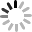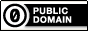## Application Information# Interactive association matrixtaendo
3

Rating

1

### Application• Play
• JavaScript
• CSS
• HTML
• SnapshotsInteractive association matrix

HOW TO USE

Left diagram indicate clustered elements calculated using cross table given as LinkData RDF.

Each cell is colored with from blue to red corresponding high odds ratio and low odds ratio.  The odds ratio is used as the distance of two elements in clustering.

The diagram responds to your cursor move and Venn diagram would be shown in right panel indicating statistical significance and raw odds ratio. P-values were obtained using hypergeometric distribution.

Preferred Venn diagrams can be saved when a cell is clicked to compare with overlap of other elements.

DATA FORMAT

The application is capable to interpret RDF tables containing cross tables.

Each row should have a 2x2 contingency table. Suppose we have two elements X and Y and sets of X-positive/-negative and Y-positive/-negative items, the contingency table follows.

Y-positive   Y-negative

X-positive    N11            N12

X-negative   N21            N22

Then the row describing the correlation is

X  Y  N11 N12 N21 N22.

If you have M elements and would like to obtain their diagram, your LinkData table should have M x (M - 1) / 2 rows.

 Contributortaendo 5852 Sep 17, 2012 Sep 26, 2012

### Forked (Child App)

forked:Interactive association matrix Contributor: David_Gifford Update: Feb 7, 2013 175 runs
forked:Interactive association matrix Contributor: taendo Update: Sep 25, 2012 141 runs
• LinkData work list used as input data in this application.
• You can change input data and execute application if you change the checkbox.

﻿